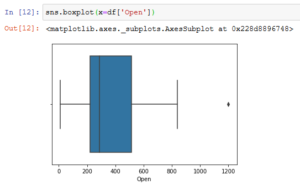# Boxplot : The most useful graphPosted by

## Introduction to Boxplot:

When we have a numeral dataset and we do not know from where to start! Boxplot can be the answer. It is like a storyteller for numerical data. It shows five essential statical measures in a single look. For example, it shows the outlier, it shows how the data is distributed per inter-quartile range, it also shows at what distance the outliers are present. Therefore, a boxplot can reveal lots of insight for any given feature.

The figure below is an example of boxplot of a normally distributed data:## What we can infer from the boxplot?

It shows the distribution of data(feature/column) with the help of a box and whisker(a perpendicular line at both the end of the box).

1. We can say it is another form of distribution graph.
2. Boxplot provides a five-point summary which helps users to get critical information about the data.
3. The five-point summary includes a minimum value, lower quartile (Q1), median value (Q2), upper quartile (Q3), maximum value.
4. Whishkers are the horizontal line that are extended from the Q1 and Q2 respectively.
5. It can be used to detect outliers.
6. Any data point outside of the whiskers is an outlier.
7. Length of the whiskers calculated as 1.5* inter-quartile range(IQR).
8. Formulation of IQR=Q3-Q1. Therefore,  this is also the length of the box.
9. Data points that are red marked are the outliers, as these data points exceed the length of the whiskers.
10. If data point more/less than 3*I.Q.R, then it is an outlier.

Enough of theory, let’s move to code…..

The code below generates a box plot using random data. Therefore, each run generates a different a diffenent boxplot.

```import pandas as pd
import numpy as np
import matplotlib.pyplot as plt
import seaborn as sns

df = pd.DataFrame( np.random.randn(5, 3),index=["a", "c", "e", "f", "h"], columns=["x1", "x2", "x3"] )

sns.boxplot(x=df["x1"])```

Output:Boxplot with Outlier: